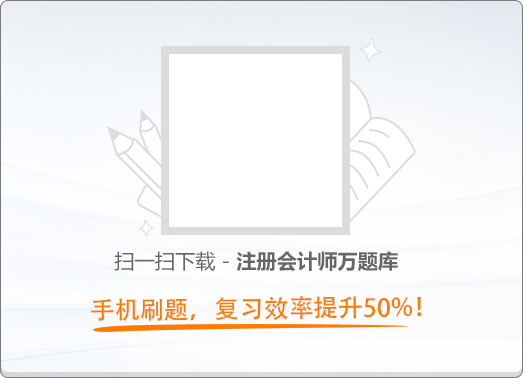120:002013年注册会计师《税法》真题

1
(单项选择题)

• A. 3750
• B. 7000
• C. 37500
• D. 70000
• A
• B
• C
• D

2
(单项选择题)

2012年1月，甲有房产原值8000万元，按照当地规定允许减除30%后按余值计税，4月将原值500万房产出租，出租期限3年，租金2万元/月，其中4-6月为免租期，甲2012年应缴纳房产税(  )万元。

• A.

64.44

• B.

65.34

• C.

65.49

• D.

66.54

• A
• B
• C
• D

3
(单项选择题)

• A. 收取快递费100元
• B. 代收快递手续费
• C. 帮助客户建立快递网络，销售快递单收取3万元
• D. 收取木框包装劳务费5万元
• A
• B
• C
• D

4
(单项选择题)

• A.

饲养家禽

• B.

远洋捕捞

• C.

海水养殖

• D.

种植中药材

• A
• B
• C
• D

5
(单项选择题)

• A.

供用电合同

• B.

融资租赁合同

• C.

人寿保险合同

• D.

法律咨询服务合同

• A
• B
• C
• D

6
(单项选择题)

• A. 车辆加装改装费
• B. 代收保险费
• C. 代收车辆牌照费
• D. 代收车辆购置税。
• A
• B
• C
• D

7
(单项选择题)

• A.

査封财产价值应缴税费的金额

• B.

通知银行代扣应缴税费的金额

• C.

拍卖资产中按照应缴税费的金额缴纳

• D.

处以5000元到20000元的罚款。

• A
• B
• C
• D

8
(单项选择题)

• A. 3.25万
• B. 9.75万
• C. 13万
• D. 32.5万
• A
• B
• C
• D

9
(单项选择题)

• A.

1.23 万

• B.

13.17 万

• C.

13.68 万

• D.

13.86 万

• A
• B
• C
• D

10
(单项选择题)

• A. 税务约谈要经所在税源管理部门批准并事先发出《税务约谈通知书》，提前通知纳税人
• B. 税务约谈的对象限于企业财务会计人员
• C. 发现外商投资企业和外国企业与其关联企业之间有关联方转让定价的问题，需要调查核实的，应移交上级税务机关国际税收管理部门(或有关部门)处理
• D. 发现纳税人有偷税等需要立案査处的税收违法行为嫌疑的，要移交税务稽査部门处理
• A
• B
• C
• D

11
(单项选择题)

• A.

儿子交契税3.6

• B.

好友交契税3

• C.

刘某交营业税6

• D.

刘某交营业税5

• A
• B
• C
• D

12
(单项选择题)

• A.

61000 元

• B.

71666.67 元

• C.

74333.33 元

• D.

75333.33 元

• A
• B
• C
• D

13
(单项选择题)

• A. 占用菜地开发花圃
• B. 占用农村集体所有的土地种植花卉
• C. 占用已成立四年的食品加工厂用地
• D. 占用鱼塘建房
• A
• B
• C
• D

14
(单项选择题)

• A. 60日内
• B. 20日内
• C. 10日内
• D. 30日内
• A
• B
• C
• D

15
(单项选择题)

• A.

0.36

• B.

0.72

• C.

1.08

• D.

1.44

• A
• B
• C
• D

16
(单项选择题)

• A.

程序优于实体原则

• B.

特别法优于普通法原则

• C.

实体从旧、程序从新原则

• D.

法律不溯及既往原则

• A
• B
• C
• D

17
(单项选择题)

• A. 17142.86
• B. 19285.71
• C. 20142.86
• D. 20250.00
• A
• B
• C
• D

18
(单项选择题)

• A. 0.5
• B. 1.2
• C. 1
• D. 2
• A
• B
• C
• D

19
(单项选择题)

• A. 15750 元
• B. 17640 元
• C. 1168.50 元
• D. 15690 元
• A
• B
• C
• D

20
(单项选择题)

• A.

劳务发生地

• B.

劳务发生地或其机构所在地

• C.

居住地或劳务发生地

• D.

其机构所在地或居住地

• A
• B
• C
• D

21
(单项选择题)

• A.

土地增值税

• B.

消费税

• C.

营业税

• D.

增值税

• A
• B
• C
• D

22
(单项选择题)

• A. 卷烟生产企业将自产的烟丝用于连续生产卷烟
• B. 化妆品厂将自产的化妆品作为样品在展销会上推广
• C. 汽车厂将自产的小汽车改装成大货车
• D. 地板生产企业将自产的实木地板用于本企业办公室的装修
• A
• B
• C
• D

23
(单项选择题)

• A.

人造原油

• B.

煤层瓦斯

• C.

卤水

• D.

洗选煤

• A
• B
• C
• D

24
(单项选择题)

• A.

5日

• B.

7日

• C.

10日

• D.

15日

• A
• B
• C
• D